### IMO Shortlist 2013 problem G1

Kvaliteta:
Avg: 3.0
Težina:
Avg: 6.0
Let$ABC$ be an acute-angled triangle with orthocenter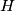$H$, and let$W$ be a point on side$BC$. Denote by$M$ and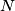$N$ the feet of the altitudes from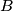$B$ and$C$, respectively. Denote by$\omega_1$ the circumcircle of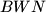$BWN$, and let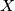$X$ be the point on$\omega_1$ which is diametrically opposite to$W$. Analogously, denote by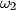$\omega_2$ the circumcircle of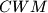$CWM$, and let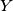$Y$ be the point on$\omega_2$ which is diametrically opposite to$W$. Prove that$X$,$Y$ and$H$ are collinear.
Source: Thailand